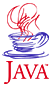Home : Map : Chapter 4 : Java : Tech : Physics : Runge-Kutta 4th Order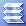Introduction Inheritance   Demo 1 Overriding   Demo 2a   Demo 2b this,super MoreConstructors   Demo 3 Abstraction Interface    Demo 4 Casting References MoreAboutArrays Object class Class Summary Exercises
 Supplements Override/Overload Annotation-J2SE5.0 Java Security Class Loading Class Verifier SecurityManager
 About JavaTech      Codes List      Exercises      Feedback      References      Resources      Tips      Topic Index      Course Guide      What's New

A further refinement of the Runge-Kutta approach uses derivatives at the beginning of the interval, at the end of the interval and twice at the midpoint.

Using the conventional k# variable names, we obtain the following increments in the variable xn with :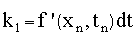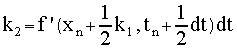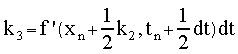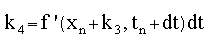Then the new increment in variable x is calculated as a weighted average of these estimated increments with k2 and k3 , the two midpoint values, given double weights.The code below shows a class created to carry out the 4th order Runge-Kutta algorithm.

 RungeKutta4th.java /**   * Provides a static method to calculate the 4th order   * Runge-Kutta integration calculation.  **/ public class RungeKutta4th {   /**     * Calculated a step of the integration of an ODE with     * 4th order RK.     *     * @param t  independent variable     * @param dt step in the independent variable     * @param var array holding the dependent variable     * @param vel array holding the first derivative     *    **/   public static void step (double t, double dt,                    double [] var,                    double [] vel,                    Derivable func ) {     double k1_var, k1_vel;     double k2_var, k2_vel;     double k3_var, k3_vel;     double k4_var, k4_vel;     for (int i=0; i < var.length; i++) {       k1_var = vel[i] * dt;       k1_vel = func.deriv (i,var[i],vel[i],t)*dt;       k2_var =  (vel[i] + 0.5*k1_vel)*dt;       k2_vel = func.deriv (i,var[i] + 0.5*k1_var,                          vel[i] + 0.5*k1_vel,                               t+0.5*dt)*dt;       k3_var =  (vel[i] + 0.5*k2_vel)*dt;       k3_vel = func.deriv (i,var[i] + 0.5*k2_var,                          vel[i] + 0.5*k2_vel,                               t+0.5*dt)*dt;       k4_var =  (vel[i] + k3_vel)*dt;       k4_vel = func.deriv (i,var[i] + k3_var,                       vel[i] + k3_vel,                            t+dt)*dt;       var[i] = var[i] +                  (k1_var + 2.0*k2_var                        + 2.0*k3_var + k4_var)/6.0;       vel[i] = vel[i] +                  (k1_vel + 2.0*k2_vel                        + 2.0*k3_vel + k4_vel)/6.0;     }     t += dt;   } // step } // RungeKutta4th

The example below is the same as previously except that the above code for the 4th order Runge-Kutta is used to the step calculation.

RK4thApplet6.java
(Output goes to browser's Java console.)

/** Program using the second order Runge-Kutta method
* to solve for the landing point of a projectile.**/
public class RK4thApplet extends java.applet.Applet
implements Derivable
{
// Constants
double g  = 9.80;// meter per sec**2
double x0 = 0.0;
double y0 = 0.0;
double v0 = 100.0;// initial vel, m/s
double angle = Math.PI/4.0;

// Instance variables
double [] pos = new double;
double [] vel = new double;

/** Set up for the algorithm. **/
public void init () {

double t;
double totalT;

int n;

double dt =  0.01;
int nSteps = 100000;

pos = x0;
pos = y0;
vel = v0 * Math.cos (angle);
vel = v0 * Math.sin (angle);
t = 0.0;

for ( n=0; n< nSteps; n++) {
RungeKutta4th.step (t,dt,pos,vel,this);
if (pos <= 0.0) break;
}

System.out.println ("After steps = " + n);
System.out.println (" x = " + pos);

} // init

/** Implement the interface method for the derivative
*  of the variables. **/
public double deriv (int i, double var, double vel, double t) {
if (i == 0) { // x variable
return 0.0;
} else {// y variable
return -g;
}
} // deriv

/** Paint message in Applet window. **/
public void paint (java.awt.Graphics g) {
g.drawString ("RK4thApplet",20,20);
} // paint

/** Run optionally as a application. **/
public static void main (String [] args) {
RK4thApplet obj = new RK4thApplet ();
obj.init ();
} // main

} // RK4thApplet

The output shows

 After steps =1443    x = 1021.0621920333822

This is the same as for the previous example since constant term for the acceleration results in the same increment to x as with the 2nd order code.

Most recent update: Oct. 21, 2005

 Tech MoreComplexClass ImprovedHistogram JavaRandomNums Vectors & Matrices Exercises
 Physics Runge-Kutta 2nd   Demo 1 Runge-Kutta 4th   Demo 2 BoundaryVal.Prob Shooting Method   Demo 3 ExercisesPart I Part II Part III Java Core 1  2  3  4  5  6  7  8  9  10  11  12 13 14 15 16 17 18 19 20 21 22 23 24 Supplements 1  2  3  4  5  6  7  8  9  10  11  12 Tech 1  2  3  4  5  6  7  8  9  10  11  12 Physics 1  2  3  4  5  6  7  8  9  10  11  12

Java is a trademark of Sun Microsystems, Inc.